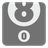The Most Numbers
Elementary+Let's work with numbers!

You are given an array of numbers (floats). You should find the difference between the maximum and minimum element. Your function should be able to handle an undefined amount of arguments. For an empty argument list, the function should return 0.

Floating-point numbers are represented in computer hardware as base 2 (binary) fractions. So we should check the result with ±0.001 precision.
Think about how to work with an arbitrary number of arguments.

Input: An arbitrary number of arguments as numbers (int, float).

Output: The difference between maximum and minimum as a number (int, float).

Example:

```mostNumbers(1, 2, 3) == 2
mostNumbers(5, -5) == 10
mostNumbers(10.2, -2.2, 0, 1.1, 0.5) == 12.4
mostNumbers() == 0
```

How it is used: Here you will learn about passing an undefined amount of arguments to functions.

Precondition: 0 ≤ len(args) ≤ 20
all(-100 < x < 100 for x in args)
all(isinstance(x, (int, float)) for x in args)

Your vote gains more value as you grow in level. Starting at level 5 you can upvote a publication twice and starting at level 14, you can upvote something five times!